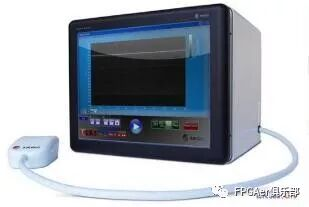2017-01-11 21:41:22 sinat_27614619 阅读数 1049
• ###### MATLAB图像处理

MATLAB图像处理课程

5054 人正在学习 去看看 李立宗

ColorDetector.h

#pragma once
#include<opencv2\opencv.hpp>
#include<iostream>
class ColorDetector
{
private:
cv::Mat m_converted;//转换颜色空间的图片
int m_threshod;//容忍度偏差
cv::Vec3b m_model;//要查找的颜色
public:
cv::Mat m_result;//最终得到的二值图像
ColorDetector();
~ColorDetector();
void setModel(cv::Vec3b &target);//设置要查找的颜色

void setModel(uchar red, uchar green, uchar blue);//设置查找颜色的接口，这种颜色值设置在RGB通道上

void setModel2(uchar red, uchar green, uchar blue);//设置查找颜色的接口，这种颜色值转换到LAB颜色空间上

void setThreshold(int distance);//设置容忍度的接口

int getDistance(cv::Vec3b &pixls);//解算图像像素点与要查找的颜色的距离

cv::Mat process(const cv::Mat &image);
cv::Mat process2(const cv::Mat &image);
};
ColorDetector.cpp
#include "ColorDetector.h"
//构造函数，给成员变量初始化，容忍度偏差设为100，查找的三通道颜色默认设为0
ColorDetector::ColorDetector():m_threshod(100)
{
m_model = m_model = m_model = 0;
}
ColorDetector::~ColorDetector()
{
}
void ColorDetector::setModel(cv::Vec3b & target)
{
m_model = target;//cv::Vec3b类型，三通道
}
void ColorDetector::setModel(uchar red, uchar green, uchar blue)
{
m_model = blue;
m_model = green;
m_model = red;
}
void ColorDetector::setModel2(uchar red, uchar green, uchar blue)
{
cv::Mat tmp(1, 1, CV_8UC3);//定义一个三通道的像素点
tmp.at<cv::Vec3b>(0, 0) = blue;
tmp.at<cv::Vec3b>(0, 0) = green;
tmp.at<cv::Vec3b>(0, 0) = red;
cv::cvtColor(tmp, tmp, CV_BGR2Lab);//颜色空间转换，把RGB——>Lab
m_model = tmp.at<cv::Vec3b>(0, 0);
}
void ColorDetector::setThreshold(int distance)
{
m_threshod = distance;//设置容忍度
}
//这里的距离公式采用1范数
int ColorDetector::getDistance(cv::Vec3b & pixls)
{
return abs(pixls - m_model)
+ abs(pixls - m_model)
+ abs(pixls - m_model);
}
cv::Mat ColorDetector::process(const cv::Mat & image)
{
m_result.create(image.size(), CV_8U);
cv::Mat_<cv::Vec3b>::const_iterator it = image.begin<cv::Vec3b>();
cv::Mat_<cv::Vec3b>::const_iterator itend = image.end<cv::Vec3b>();
cv::Mat_<uchar>::iterator out = m_result.begin<uchar>();
while (it != itend)
{
//循环遍历，把查找到的颜色转换为二值图像，这里按照RGB空间的颜色距离解算
if (getDistance(*it) < m_threshod)
*out = 255;
else
*out = 0;
++it;
++out;
}
return m_result;
}
cv::Mat ColorDetector::process2(const cv::Mat & image)
{
m_result.create(image.size(), CV_8U);
m_converted.create(image.size(), image.type());
//把输入图像从RGB颜色空间转换到Lab颜色空间
cv::cvtColor(image, m_converted, CV_BGR2Lab);
cv::Mat_<cv::Vec3b>::const_iterator it = m_converted.begin<cv::Vec3b>();
cv::Mat_<cv::Vec3b>::const_iterator itend = m_converted.end<cv::Vec3b>();
cv::Mat_<uchar>::iterator out = m_result.begin<uchar>();
while (it != itend)
{
if (getDistance(*it) < m_threshod)
*out = 255;
else
*out = 0;
++it;
++out;
}
return m_result;
}

main.cpp
#include"ColorDetector.h"
int main()
{

ColorDetector detector;//构造类

detector.setModel(130, 190, 230);//查找蓝天的颜色
cv::namedWindow("Process");
cv::imshow("Process", detector.process(image));
detector.setModel2(130, 190, 230);//查找蓝天的颜色
cv::namedWindow("Process2");
cv::imshow("Process2", detector.process2(image));

cv::waitKey(0);
return 0;
}2014-01-13 21:50:00 xj2419174554 阅读数 5695
• ###### MATLAB图像处理

MATLAB图像处理课程

5054 人正在学习 去看看 李立宗

# 2.程序设计思路

1）、直方图灰度分布均衡化去雾算法

A.统计每个灰度等级对应的像素点数

B.计算累计概率

C.重新映射图像像素点灰度上述坐标图和积分式解释了积分思想求累计概率的正确性。下面举个例子展示整个过程。

a.假设下面使是图像对应的像素点：b.下面是中间处理工程c.经过处理后图像对应的像素点：综上，直方图灰度分布均衡化去雾算法可以处理灰色图，但对于彩色图像就会出现走样，达不到去雾的效果。为了解决彩色图像的去雾问题，我又看了一些最新论文，其中包括何凯明博士的《Single Image Haze Removal Using Dark Channel Prior》论文，这是一篇非常深刻的文章，里面公式推导很多，我硬着头皮读完后看不懂，于是又到博客园、CSDN上筛选出几篇相对容易理解和实现的论文，其中我对清华大牛刘倩、陈茂银、周东华的《基于单幅图像的快速去雾算法》论文非常感兴趣，决定以其为突破点尝试彩色图像的去雾算法实现。

2.基于单幅图像的快速去雾算法

x为空间坐标，F是待恢复的无雾图像，r是大气散射系数，d是景物深度，A是与x无关的全局大气光，H为有雾情况下的景物图像。上面是程序的算法流程，下面我将依次谈谈我对每个步骤的理解。

Step 1  读入待处理的图像

Step 2 求空间最小颜色通道

Step 3 M进行均值滤波

均值滤波是徐老师在课堂上重点讲过的。均值滤波是典型的线性滤波算法，它是指在图像上对目标像素给一个模板，该模板包括了其周围的临近像素，再用模板中的全体像素的平均值来代替原来像素值。 可见均值滤波的关键是选择模板，然后对应相乘相加相除，这个过程是比较简单。

Step 4 M所有像素点求均值Mav

Step 5  求环境光估计值

Step 6 求出全局大气光

Step 7 还原图像

考虑环境光、全局大气光的影响，利用算法开头给出的模型表达式还原图片。

上面就是整个过程。在原论文中，上述都给出较为严格的证明，由于相关专业知识有限，我就不交代推理证明过程了。

# 3.数据结构与算法设计

1）、直方图灰度分布均衡化去雾算法

由于整个处理过程中没有特殊要求的操作，所以开辟二维数组就可解决问题。分别开辟3个二维数组

int *Color=new int[MAXNCOLOR];//Color[i]存储该灰度等级i对应的像素点数，同时为了节省内存空间，在映射后Color[i]则存储原来灰度等级i对应的新灰度等级，这样做可以少申请一个二维数组，由于图像处理很占内存空间，因此优化是必须的。

double *PerProbability=new double[MAXNCOLOR];//存储灰度等级i对应的概率PerProbability[i].

double* AccuProbability=new double[MAXNCOLOR];//存储灰度等级i对应的累加概率AccuProbability[i],此数组可以和上面的PerProbability[]数组合成一个，为了程序的可读性，我开了两个数组，但须明确此处可以优化。

2.基于单幅图像的快速去雾算法

上面的空间最小颜色通道和均值Mav可以在求所有点的颜色通道是一起求出，不必另写循环语句比较求得。由于编程实现较简单，在此就不多说了。

具体算法步骤见程序设计思路部分，具体源代码见第4部分基于单幅图像的快速去雾算法。

# 4.程序关键代码

```、直方图灰度分布均衡化去雾算法源代码
/*************************************************
*功能：直方图灰度分布均衡化去雾算法
**************************************************/
{
const int MAXNCOLOR=256+10;
unsigned char *lpSrc;
CMyDIPDoc* pDoc = GetDocument();
ASSERT_VALID(pDoc);
if(pDoc->m_hDIB == NULL)
return ;
LPSTR lpDIB = (LPSTR) ::GlobalLock((HGLOBAL) pDoc->m_hDIB);
LPSTR lpDIBBits=::FindDIBBits (lpDIB);
int cxDIB = (int) ::DIBWidth(lpDIB);
int cyDIB = (int) ::DIBHeight(lpDIB);
int *Color=new int[MAXNCOLOR];
memset(Color,0,sizeof(Color));
long lLineBytes = WIDTHBYTES(cxDIB * 8);

//统计每个灰度等级对应的像素点数
for(int i = 0; i < cyDIB; i++)
{
for(int j = 0; j < cxDIB; j++)
{
Color[*(lpDIBBits + lLineBytes * (cyDIB - 1 - i) + j)]++;
}
}

//计算每个灰度等级对应的概率
double *PerProbability=new double[MAXNCOLOR];
double TotalPixelNumber=cxDIB*cyDIB*1.0;
for(i=0;i<256;i++)
{
PerProbability[i]=Color[i]*1.0/TotalPixelNumber;
}
//计算每个灰度等级对应的累加概率
double* AccuProbability=new double[MAXNCOLOR];
AccuProbability=PerProbability;
Color=(int)(AccuProbability*255.0f+0.5f);
for(i=1;i<256;i++)
{
AccuProbability[i]=AccuProbability[i-1]+PerProbability[i];
Color[i]=(int)(AccuProbability[i]*255.0f);
}

//重新映射屏幕像素点灰度
for(i = 0; i < cyDIB; i++)
{
for(int j = 0; j < cxDIB; j++)
{
unsigned char perpixel=*(lpDIBBits + lLineBytes * (cyDIB - 1 - i) + j);
*(lpDIBBits + lLineBytes * (cyDIB - 1 - i) + j)=Color[perpixel];

}
}
::GlobalUnlock((HGLOBAL) pDoc->m_hDIB);
Invalidate(TRUE);
}

.基于单幅图像的快速去雾算法
/*************************************************
*功能：基于单幅图像的快速去雾算法
**************************************************/
{
unsigned char *lpSrc;
CMyDIPDoc* pDoc = GetDocument();
ASSERT_VALID(pDoc);
if(pDoc->m_hDIB == NULL)
return ;
LPSTR lpDIB = (LPSTR) ::GlobalLock((HGLOBAL) pDoc->m_hDIB);
LPSTR lpDIBBits=::FindDIBBits (lpDIB);
int cxDIB = (int) ::DIBWidth(lpDIB);
int cyDIB = (int) ::DIBHeight(lpDIB);
long lLineBytes = WIDTHBYTES(cxDIB * 8);
double sumMinColor=0;//所有颜色通道的累加和
double aveMinColor=0;//所有颜色通道的平均值
unsigned char minColor;//颜色通道最小值
unsigned char maxColor=0;//所有颜色通道最大值
int maxFlag=0;//标记颜色通道最大值权重
int minFlag=0;//标记颜色通道最小值权重
unsigned char *mNewPicture=new unsigned char[cxDIB*cyDIB];
for(int i = 0; i < cyDIB; i++)
{
for(int j = 0; j < cxDIB; j++)
{

unsigned char perpixel=*(lpDIBBits + lLineBytes * (cyDIB - 1 - i) + j);
unsigned char red=perpixel/32;
minFlag=32;
minColor=red;
if(maxColor<red)
{
maxColor=red;
maxFlag=32;
}
perpixel%=32;
unsigned char green=perpixel/8;
if(minColor>green)
{
minColor=green;
minFlag=8;
}
if(maxColor<green)
{
maxColor=green;
maxFlag=8;
}

unsigned char blue=perpixel%8;
if(minColor>blue)
{
minFlag=1;
minColor=blue;
}
if(maxColor<blue)
{
maxColor=blue;
maxFlag=1;
}
mNewPicture[cxDIB * (cyDIB - 1 - i) + j]=minColor*minFlag;
sumMinColor+=minColor*minFlag;
}
}
aveMinColor=sumMinColor/(cxDIB*cyDIB);//颜色通道平均值
maxColor*=maxFlag;
double maxAveColor=0;//均值滤波后颜色通道平均值
double *mAveNewPicture=new double[cxDIB*cyDIB];
double xishu=1.0/100.0;
int h=cyDIB,w=cxDIB;
int tem_w=10,tem_h=10;
int dw = cxDIB;
double sum;
for(i=0;i<h;i++)
{
for(int j=0;j<w;j++)
{
sum=0;
if( j<((tem_w-1)/2) || j>(w-(tem_w+1)/2) || i<((tem_h-1)/2) || i>(h-(tem_h+1)/2) )
mAveNewPicture[i*dw+j]=mNewPicture[i*dw+j];
else
{
for(int m=i-((tem_h-1)/2);m<=i+((tem_h-1)/2);m++)
{
for(int n=j-((tem_w-1)/2);n<=j+((tem_w-1)/2);n++)
sum+=mNewPicture[m*dw+n];

}
sum=sum*xishu;sum = fabs(sum);
if(sum<0)     sum=0;
if(sum>255)sum=255;
mAveNewPicture[i*dw+j]=sum;
}
if(maxAveColor<mAveNewPicture[i*dw+j])
maxAveColor=mAveNewPicture[i*dw+j];
}
}
double *lNewPicture=new double[cxDIB*cyDIB];
for(i = 0; i < cyDIB; i++)
{
for(int j = 0; j < cxDIB; j++)
{
lNewPicture[i*cxDIB+j]=min(min(aveMinColor*1.28,0.9)*mAveNewPicture[i*cxDIB+j],mNewPicture[i*cxDIB+j]);
}
}
double A=(maxColor+maxAveColor)/2.0;
for(i = 0; i < cyDIB; i++)
{
for(int j = 0; j < cxDIB; j++)
{
double H=*(lpDIBBits + lLineBytes * (cyDIB - 1 - i) + j);
double L=lNewPicture[cxDIB * (cyDIB - 1 - i) + j];
*(lpDIBBits + lLineBytes * (cyDIB - 1 - i) + j)=(H-L)/(1-L/A);
}
}
::GlobalUnlock((HGLOBAL) pDoc->m_hDIB);
Invalidate(TRUE);
}```

# 5.程序运行结果

（2）、直方图灰度分布均衡化去雾算法

去雾前去雾前：对于上面3张灰度图像，直方图灰度分布均衡化达到的去雾效果是不错的，但是对于彩色图像，却无法回避走样问题。下面给出的就是直方图灰度分布均衡化在处理彩色图像时遇到的问题。

去雾前图像4在直方图灰度分布均衡化后景物形状保持了，但色彩却严重偏离了，关于原因，上面已有解释。

（2）.基于单幅图像的快速去雾算法去雾前去雾前# 6.编程中遇到的困难及解决方法

1）、选题

由于徐老师没有要求具体做什么，这个长期习惯老师出题然后自己动手编程的我带来一定干扰。自主选题也是一种自主学习能力，结合自己的兴趣与能力选择适合自己的选题。由于之前做ACM，我认为自己有一定的解决问题能力，于是选择了较为前沿的去雾算法。

（2）、查资料

（3）、关于bmp格式的256色位图的处理

（4）、直方图灰度分布均衡化去雾算法处理彩色图

《限制对比度自适应直方图均衡化算法原理、实现及效果》以及

《局部自适应自动色阶/对比度算法在图像增强上的应用》文章发现用人工智能中自适应方法也可以使直方图均衡化具备处理彩色图像的能力。但自适应的相关理论对刚开始接触图像处理的我来说有难度，所以我只用直方图均衡化处理灰度图像。

（5）、获取bmp格式的256色位图的颜色通道

（6）、均值滤波模板大小的设置

关于这点，徐老师课堂上是可以结合实际情况自己设定。但当面对具体情况时，模板大小的设定很有技巧。均值滤波可以对图像进行模糊处理、平滑处理，在一定范围了随模板大小的增大而增大，当达到某个峰值之后随模板大小的增大而减小。上述是本次实验经验所得，可能不符合理论，但我的程序结果却支持这种结论。

（7）、ρ参数的大小设置

ρ参数的大小控制了图像去雾能力的大小，越大，雾气越少，图像越显得暗，越小，图像偏白，雾气越浓。所以在合适的范围内参数ρ应该尽可能取得大点，实验中我去的是1.28

# 7.总结心得

在本次写报告的过程中，我最大的感觉就是知识欠缺。在我目前接触的图像处理算法中，没有特别难编程实现的，大多可以通过基本的编程方法解决。但是，其中的指导思想却是很需要用心体会的。在编程时一定要上升到理论层次，多问几个为什么：为什么这么做，为什么会有这样的效果等等。

在我看来，图像处理就是一个矩阵变换，变换的是矩阵中对应空间坐标的像素值。而变换过程中要用到很多模板、算子、算法等，这些是进行矩阵变换的依据。在理论工作完成以后，再进行动手编程，这样才能达到真正学习图像处理与分析的目的。有的理论看起来很简单，但却温含着丰富的专业知识，需要我们静下心来慢慢体会，仔细琢磨；有的理论看起来就很难，就像我选的《基于单幅图像的快速去雾算法》这篇论文，从头到尾都体现着丰富的专业知识、严密的逻辑推理能力与深厚的科学内涵，对于这样的理论，更是难得的思想启蒙，我们应该迎难而上，尽可能的读懂它。当然在编程的过程中，我们也要不断优化自己的程序，图像对象的数据量很大，稍不留神算法的时间或空间复杂度就会很高，即便常数级的优化也是很有必要的，能少开辟数组是就少开辟，能少用一层循环就少用一层循环。

8、参考文献

1.刘倩、陈茂银、周东华 《基于单幅图像的快速去雾算法》论文

2.CSDN博客兰专家laviewpbt博客《一种可实时处理 O(1)复杂度图像去雾算法的实现

3.何凯明 《Single Image Haze Removal Using Dark Channel Prior》论文

如果觉得对你有所帮助，给几毛资助也是不错的想法！下面是博主的支付宝二维码，如果有一天收到你的资助，将无比欣慰！2015-05-09 18:18:20 luoweifu 阅读数 1128
• ###### MATLAB图像处理

MATLAB图像处理课程

5054 人正在学习 去看看 李立宗

## 《数据结构(java)》

2014-07-29 18:45:23 iloveas2014 阅读数 605
• ###### MATLAB图像处理

MATLAB图像处理课程

5054 人正在学习 去看看 李立宗

## 内容编排

本套教程共分3部分。

第一部分介绍色彩的相关知识及Flash，Photoshop等可视化软件在图形图像处理方面的一些特性，包括常用的色彩模式，Photoshop图层效果，ActionScript滤镜，混合模式等知识，并指出这些特性在不同软件下的异同。

第二部分以应用数学为思想，以BitmapData，像素为工具，深入剖析各种图像处理技术的工作原理，让读者可以深入到算法的层面上认识图像处理，在ActionScript乃至其它支持像素级编程的语言中实现与Photoshop等专业图像软件相媲美的图形效果。

第三部分将充分发挥程序在交互、随机等方面的优势，运用以上学过的知识制作各种有趣的应用，游戏或者交互动画效果，让您的程序作品以艺术的形式走进千家万户，感染着与您互动的每一位观众。

2018-05-27 22:38:12 FPGAerClub 阅读数 3827
• ###### MATLAB图像处理

MATLAB图像处理课程

5054 人正在学习 去看看 李立宗============华 丽 的 分 割 线============﻿﻿